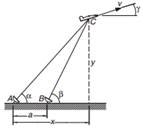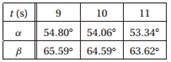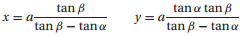Create an Account

Home / Questions / The radar stations A and B separated by the distance a 500 m track the plane C by record...

The radar stations A and B separated by the distance a 500 m track the plane C by recording the angles α and β at one second intervals If three successive readings are calculate the speedThe radar stations A and B, separated by the distance a = 500 m, track the plane C by recording the angles α and β at one-second intervals. If three successive readings arecalculate the speed v of the plane and the climb angle γ at t = 10 s. The coordinates of the plane can be shown to beJul 27 2020 View more View LessSubscribe To Get Solution